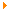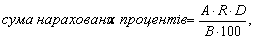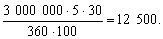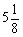This page has been robot translated, sorry for typos if any. Original content here.

 homeOther disciplinesBooksInternational markets and foreign exchange operations - Savluk M.І.

# International markets and foreign exchange operations - Savluk M.І.

## 9.3. Interest rates on the market of interbank deposits in foreign currencies

Interest rate - the price of resources. There are rozmishchuyuchi resources in the penny market, where the hair owners get the most out of their money, besides, the fee for the payment of resources includes:

• Compensation for credit loans, which should be considered as a credit operation, rozmir reimbursement of indemnity to lay down the class of the principal;
• Compensation for indefinite likelihood: less insignificant pozik to rely on the minds “before eating” and to turn on the feathers at the most, the main part of the cost is better to not have more problems;
• compensation for contributions hour of gain in income: interest for the loan is paid in the most part, the payment term is higher, the bank is able to quickly receive the money, the interest rate is higher.

Razrіznyayut nominal and real interest rates. The real interest rate is the nominal rate (that is, the rate that was placed on the market), adjusted for the percentage of inflation. For example, if the nominal rate for a deposit becomes - 8% of the average, and the inflation is 6%, then the real interest rate is 2%.

Riven of market interest rates can be given by formula * 1:

* 1: {In order to describe the ranks of the Fischer Effects, which means that the loan is between the nominal and the real interest rates and the correct interest rate.}

k = r + x * + p + m,

de k - rіven interest rates scho sposterіgaєtsya at the market;

r - real interest rate;

x * - ochuvivaniy riven іnflatsyї

p - premium (premium) for credit riser, tobto for riser vtrat through non-trust koshtіv;

m - premium (premium) for the interest rate interest rate, which is the benefit of interest rates for the interest rate.

The real interest rate and the inflation rate at once signify the nominal rate of the interest rate risk ( i = r + x ).

Sounds:

k = i + p + m.

On the whole, there is a risk of interest rate percentage, higher inflation rate and lower interest rate, lower inflation rate:

• a penny-credit policy of the central bank : the policy of “expensive pennies”, is fixed on the basis of the pennies of the penny, drag on itself the growth of the interest rate and the policy of the “cheap pennies” to lower the interest rates;
• consume credit resources to recognize interest rates on state fixed prices, which can be used as an alternative to costly money (in countries with a low interest rate, lower costs are important): Then, at your own place, you can pay attention to commercial banks with interest rates and interest rates. At the same time, if the demand for cats decreases, the interest rates for the sovereign valued daddies decrease, so as to cause lower interest rates on the market;
• payment balance of the country . Interest rates є one of the tools to cover the capital items of the balance of payments: the policy is straightforward, fixed on the basis of foreign capital, if you are supervised by the central bank of interest rates;
• drank to a penny market , as well as a rush in economic activity : more income, more characteristic for a period of more economical activity, higher interest rates, a lower recession, which is more important;
• ochіkuvannya on market analysis: Yakscho ochіkuєtsya padіnnya interest rates, the Ti Participants fіnansovogo market analysis, scho toil vіlnі resources will namagatisya rozmіstiti їh on bіlsh trivaly termіn, dwellers will win the maximum Prybutok od TEMPLE rates when rozmіschennya koshtіv, ale pozichalniki have their Cherga, zaluchatimut short-term deposits, ochuyuchi, if the rates are lowered. In the result of pre-term interest rates, it will fall into just short lines. If you want to increase interest rates, you will drink on pre-term poses to get better, for those who want to get more interest, you can lower rates, water lenders will be able to lower the pre-term interest rate;
• rіven interest rates svіtovih fіnansovih Rink: іnternatsіonalіzatsіya fіnansovih rinkіv that chastkove abo Povny skasuvannya bіlshіstyu rozvinutih kraїn obmezhen on zdіysnennya konversіynih operatsіy spriyayut fact scho pіdvischennya interest rates on one of rinkіv zumovlyuє spryamuvannya resursіv tudi, de stink will achieve maximum dohіd.

At the level of interest rates for deposits in foreign currency, mostly the officials will absorb the money from the side of the country that is worth the currency rather than the country, and the operation is more expensive and the cost is better.

When there is a significant amount of deposit operations in foreign currency, the interest on the basis of simple interest is:de A - sum to deposit;

R is the interest rate;

D - number of days of the interest period;

B - the basic number of days at the rosts (360 abo 365).

For most currencies, the basic number of days at the same time is the same for 360, the ale for denominated currencies (the British pound, the Irish pound, the Belgian franc, the Singapore dollar, the Hong Kong dollar and the 365 euro).

For example, interest, you need to maintain a bank with a loan of 3 million euros in a line for one month 5% of the average, expensive 12,500 euros:The interest rate is called є the primary interest rate, it is pa (the Latin per annum is на))).

In international practice, there are two ways to write the interest rate: in the view of the decimal fraction (5.125%) and the ration fraction (%).

The interest rate may be fixed or floating.

The interest rate is fixed at a fixed / recovered deposit and indemnified for the entire period of the deposit. Such a rate, please wait for short-term pleasures.

A floating interest rate can be extended by an extended deposit. The most frequent floating rates for currency market deposits are tied up to the LIBOR rate. This is the average rate, for which the most British banks lend to the first-class posters at the London Interbank Offered Rate. The rate is fixed for 11.00 year of skin day. Існує the same LIBID rate (London Interbank Bid Rate), so that the rate is the best for all banks. LIBOR rates are quoted for British pounds, US dollars, Euros, Swiss francs and Japanese ones. At LIBOR floating interest rates, the basis is victorious, basis points - bp are reached. One hundred base points are more than one percent, so 1 bp = 0.01%. For example, the bank quotes the US dollar interest rate for such a method:

USD 6m LIBOR + 75 bp (six-month LIBOR rate + 0.75%).

For US dolars, the base of the floating rate can be vikoristovatsya so called the base rate of the prime rate (prime rate). The price is for short-term commercial posits to first-class applicants, who will quote the most American banks. As if the posic in the US dollars is beyond the boundaries of the United States, then the LIBOR rate will be lavishly victorious. Also, the base can be used in the past: the FIBOR rate (Frankfurt Interbank Offered Rate), the rates of sovereign folders and commercial folders.